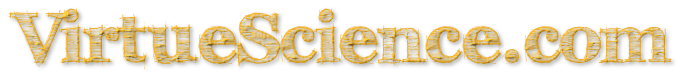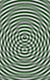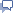<128 Number Data-BaseRandom Number130>

## The Number 129: Properties and Meanings

Prime Factors of 129=3x43.

129 can be Partitioned 65 times with each term no larger than 2.

129 can be Partitioned 1452 times with each term no larger than 3.

129 is a 44-gonal Number.

129 is the smallest number that can be written as the sum of 3 squares in 4 ways.

129 is the sum of the first ten primes.

129 is the smallest sum of distinct seventh powers.

 <128 Number Data-BaseRandom Number130>Share any properties and meanings for particular Numbers...contact me directly, thanks.